Logistic-Normal Model with Binomial Data

This example analyzes the data from Beitler and Landis (1985), which represent results from a multi-center clinical trial investigating the effectiveness of two topical cream treatments (active drug, control) in curing an infection. For each of eight clinics, the number of trials and favorable cures are recorded for each treatment. The SAS data set is as follows.

```data infection;
input clinic t x n;
datalines;
1 1 11 36
1 0 10 37
2 1 16 20
2 0 22 32
3 1 14 19
3 0  7 19
4 1  2 16
4 0  1 17
5 1  6 17
5 0  0 12
6 1  1 11
6 0  0 10
7 1  1  5
7 0  1  9
8 1  4  6
8 0  6  7
;
```

Suppose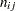denotes the number of trials for theth clinic and theth treatment (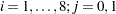), and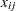denotes the corresponding number of favorable cures. Then a reasonable model for the preceding data is the following logistic model with random effects: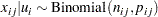and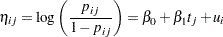The notation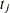indicates theth treatment, and the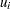are assumed to be iid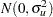.

The PROC NLMIXED statements to fit this model are as follows:

```proc nlmixed data=infection;
parms beta0=-1 beta1=1 s2u=2;
eta    = beta0 + beta1*t + u;
expeta = exp(eta);
p      = expeta/(1+expeta);
model x ~ binomial(n,p);
random u ~ normal(0,s2u) subject=clinic;
predict eta out=eta;
estimate '1/beta1' 1/beta1;
run;
```

The PROC NLMIXED statement invokes the procedure, and the PARMS statement defines the parameters and their starting values. The next three statements define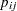, and the MODEL statement defines the conditional distribution ofto be binomial. The RANDOM statement defines u to be the random effect with subjects defined by the clinic variable.

The PREDICT statement constructs predictions for each observation in the input data set. For this example, predictions of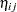and approximate standard errors of prediction are output to a data set named eta. These predictions include empirical Bayes estimates of the random effects.

The ESTIMATE statement requests an estimate of the reciprocal of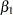.

The output for this model is as follows.

Figure 63.7 Model Information and Dimensions for Logistic-Normal Model
The NLMIXED Procedure

Specifications
Data Set WORK.INFECTION
Dependent Variable x
Distribution for Dependent Variable Binomial
Random Effects u
Distribution for Random Effects Normal
Subject Variable clinic
Optimization Technique Dual Quasi-Newton

Dimensions
Observations Used 16
Observations Not Used 0
Total Observations 16
Subjects 8
Max Obs Per Subject 2
Parameters 3

The "Specifications" table provides basic information about the nonlinear mixed model (Figure 63.7). For example, the distribution of the response variable, conditional on normally distributed random effects, is binomial. The "Dimensions" table provides counts of various variables. You should check this table to make sure the data set and model have been entered properly. PROC NLMIXED selects five quadrature points to achieve the default accuracy in the likelihood calculations.

Figure 63.8 Starting Values of Parameter Estimates
Parameters
beta0 beta1 s2u NegLogLike
-1 1 2 37.5945925

The "Parameters" table lists the starting point of the optimization and the negative log likelihood at the starting values (Figure 63.8).

Figure 63.9 Iteration History and Fit Statistics for Logistic-Normal Model
Iteration History
Iter   Calls NegLogLike Diff MaxGrad Slope
1   2 37.3622692 0.232323 2.882077 -19.3762
2   3 37.1460375 0.216232 0.921926 -0.82852
3   5 37.0300936 0.115944 0.315897 -0.59175
4   6 37.0223017 0.007792 0.01906 -0.01615
5   7 37.0222472 0.000054 0.001743 -0.00011
6   9 37.0222466 6.57E-7 0.000091 -1.28E-6
7   11 37.0222466 5.38E-10 2.078E-6 -1.1E-9

 NOTE: GCONV convergence criterion satisfied.

Fit Statistics
-2 Log Likelihood 74.0
AIC (smaller is better) 80.0
AICC (smaller is better) 82.0
BIC (smaller is better) 80.3

The "Iteration History" table indicates successful convergence in seven iterations (Figure 63.9). The "Fit Statistics" table lists some useful statistics based on the maximized value of the log likelihood.

Figure 63.10 Parameter Estimates for Logistic-Normal Model
Parameter Estimates
Parameter Estimate Standard Error DF t Value Pr > |t| Alpha Lower Upper Gradient
beta0 -1.1974 0.5561 7 -2.15 0.0683 0.05 -2.5123 0.1175 -3.1E-7
beta1 0.7385 0.3004 7 2.46 0.0436 0.05 0.02806 1.4488 -2.08E-6
s2u 1.9591 1.1903 7 1.65 0.1438 0.05 -0.8554 4.7736 -2.48E-7

The "Parameter Estimates" table indicates marginal significance of the two fixed-effects parameters (Figure 63.10). The positive value of the estimate ofindicates that the treatment significantly increases the chance of a favorable cure.

Figure 63.11 Table of Additional Estimates
The "Additional Estimates" table displays results from the ESTIMATE statement (Figure 63.11). The estimate of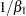equals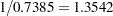and its standard error equals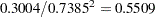by the delta method (Billingsley 1986, Cox 1998). Note that this particular approximation produces a-statistic identical to that for the estimate of.
Not shown is the eta data set, which contains the original 16 observations and predictions of the.